Courses

# Trees Computer Science Engineering (CSE) Notes | EduRev

## Computer Science Engineering (CSE) : Trees Computer Science Engineering (CSE) Notes | EduRev

The document Trees Computer Science Engineering (CSE) Notes | EduRev is a part of the Computer Science Engineering (CSE) Course GATE Computer Science Engineering(CSE) 2022 Mock Test Series.
All you need of Computer Science Engineering (CSE) at this link: Computer Science Engineering (CSE)

## Binary Tree | (Introduction)

Trees: Unlike Arrays, Linked Lists, Stack and queues, which are linear data structures, trees are hierarchical data structures.

Tree VocabularyThe topmost node is called root of the tree. The elements that are directly under an element are called its children. The element directly above something is called its parent. For example, a is a child of f and f is the parent of a. Finally, elements with no children are called leaves.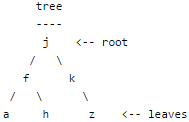Why Trees?
1. One reason to use trees might be because you want to store information that naturally forms a hierarchy. For example, the file system on a computer: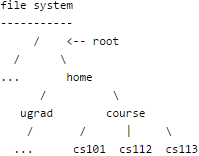2. Trees (with some ordering e.g., BST) provide moderate access/search (quicker than Linked List and slower than arrays).
3. Trees provide moderate insertion/deletion (quicker than Arrays and slower than Unordered Linked Lists).
4. Like Linked Lists and unlike Arrays, Trees don’t have an upper limit on number of nodes as nodes are linked using pointers.

Main applications of trees include:
1. Manipulate hierarchical data.
2. Make information easy to search (see tree traversal).
3. Manipulate sorted lists of data.
4. As a workflow for compositing digital images for visual effects.
5. Router algorithms
6. Form of a multi-stage decision-making (see business chess).

Binary Tree: A tree whose elements have at most 2 children is called a binary tree. Since each element in a binary tree can have only 2 children, we typically name them the left and right child.

Binary Tree Representation in C: A tree is represented by a pointer to the topmost node in tree. If the tree is empty, then value of root is NULL.
A Tree node contains following parts.
1. Data
2. Pointer to left child
3. Pointer to right child

In C, we can represent a tree node using structures. Below is an example of a tree node with an integer data.

struct node
{
int data;
struct node *left;
struct node *right;
};

First Simple Tree in C
Let us create a simple tree with 4 nodes in C. The created tree would be as following.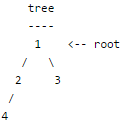struct node
{
int data;
struct node *left;
struct node *right;
};

/* newNode() allocates a new node with the given data and NULL left and
right pointers. */
struct node* newNode(int data)
{
// Allocate memory for new node
struct node* node = (struct node*)malloc(sizeof(struct node));

// Assign data to this node
node->data = data;

// Initialize left and right children as NULL
node->left = NULL;
node->right = NULL;
return(node);
}

int main()
{
/*create root*/
struct node *root = newNode(1);
/* following is the tree after above statement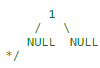root->left        = newNode(2);
root->right       = newNode(3);
/* 2 and 3 become left and right children of 1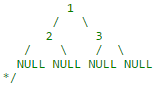root->left->left  = newNode(4);
/* 4 becomes left child of 2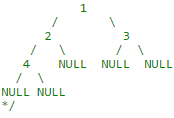getchar();
return 0;
}

Summary: Tree is a hierarchical data structure. Main uses of trees include maintaining hierarchical data, providing moderate access and insert/delete operations. Binary trees are special cases of tree where every node has at most two children.

Binary Tree |  (Properties)

We have discussed Introduction to Binary Tree. In this post, properties of binary are discussed.

1) The maximum number of nodes at level ‘l’ of a binary tree is 2l-1.

Here level is number of nodes on path from root to the node (including root and node). Level of root is 1.
This can be proved by induction.
For root, l = 1, number of nodes = 21-1 = 1
Assume that maximum number of nodes on level l is 2l-1
Since in Binary tree every node has at most 2 children, next level would have twice nodes, i.e. 2 * 2l-1

2) Maximum number of nodes in a binary tree of height ‘h’ is 2h – 1.

Here height of a tree is maximum number of nodes on root to leaf path. Height of a leaf node is considered as 1.
This result can be derived from point 2 above. A tree has maximum nodes if all levels have maximum nodes. So maximum number of nodes in a binary tree of height h is 1 + 2 + 4 + .. + 2h-1. This is a simple geometric series with h terms and sum of this series is 2h – 1.
In some books, height of a leaf is considered as 0. In this convention, the above formula becomes 2h+1 – 1

3) In a Binary Tree with N nodes, minimum possible height or minimum number of levels is  ⌈ Log2(N+1) ⌉

This can be directly derived from point 2 above. If we consider the convention where height of a leaf node is considered as 0, then above formula for minimum possible height becomes   ⌈ Log2(N+1) ⌉ – 1

4) A Binary Tree with L leaves has at least   ⌈ Log2L ⌉ + 1   levels

A Binary tree has maximum number of leaves when all levels are fully filled. Let all leaves be at level l, then below is true for number of leaves L.

L   <=  2l-1  [From Point 1]
Log2L <=  l-1
l >=   ⌈ Log2L ⌉ + 1

5) In Binary tree, number of leaf nodes is always one more than nodes with two children.

L = T + 1
Where L = Number of leaf nodes
T = Number of internal nodes with two children

Binary Tree | (Types of Binary Tree)

We have discussed Introduction to Binary Tree and Properties of Binary Tree. In this post, common types of binary is discussed.

Following are common types of Binary Trees.

Full Binary Tree A Binary Tree is full if every node has 0 or 2 children. Following are examples of full binary tree.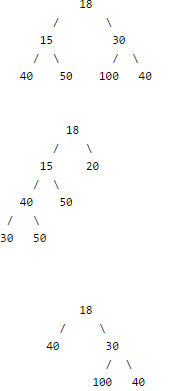In a Full Binary, number of leaf nodes is number of internal nodes plus 1
L = I + 1
Where L = Number of leaf nodes, I = Number of internal nodes

Complete Binary Tree: A Binary Tree is complete Binary Tree if all levels are completely filled except possibly the last level and the last level has all keys as left as possible

Following are examples of Complete Binary Trees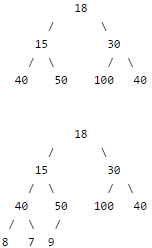Perfect Binary Tree A Binary tree is Perfect Binary Tree in which all internal nodes have two children and all leaves are at same level.
Following are examples of Perfect Binaryr Trees.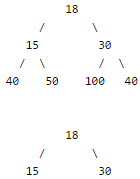A Perfect Binary Tree of height h (where height is number of nodes on path from root to leaf) has 2h – 1 node.

Example of Perfect binary tree is ancestors in family. Keep a person at root, parents as children, parents of parents as their children.

Balanced Binary Tree

A binary tree is balanced if height of the tree is O(Log n) where n is number of nodes. For Example, AVL tree maintain O(Log n) height by making sure that the difference between heights of left and right subtrees is 1. Red-Black trees maintain O(Log n) height by making sure that the number of Black nodes on every root to leaf paths are same and there are no adjacent red nodes. Balanced Binary Search trees are performance wise good as they provide O(log n) time for search, insert and delete.

A degenerate (or pathological) tree A Tree where every internal node has one child.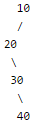## Applications of tree data structure

Difficulty LevelRookie

Why Tree?

Unlike Array and Linked List, which are linear data structures, tree is hierarchical (or non-linear) data structure.

1) One reason to use trees might be because you want to store information that naturally forms a hierarchy. For example, the file system on a computer:

file system
———–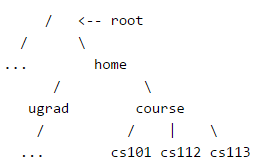2) If we organize keys in form of a tree (with some ordering e.g., BST), we can search for a given key in moderate time (quicker than Linked List and slower than arrays).

3) We can insert/delete keys in moderate time (quicker than Arrays and slower than Unordered Linked Lists).

4) Like Linked Lists and unlike Arrays, Pointer implementation of trees don’t have an upper limit on number of nodes as nodes are linked using pointers.

As per Wikipedia, following are the common uses of tree.
1. Manipulate hierarchical data.
2. Make information easy to search (see tree traversal).
3. Manipulate sorted lists of data.
4. As a workflow for compositing digital images for visual effects.
5. Router algorithms

Tree Traversals (Inorder, Preorder and Postorder)

Unlike linear data structures (Array, Linked List, Queues, Stacks, etc) which have only one logical way to traverse them, trees can be traversed in different ways. Following are the generally used ways for traversing trees.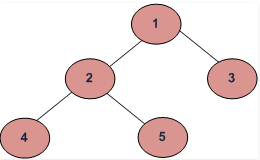Example Tree

Depth First Traversals:
(a) Inorder (Left, Root, Right) : 4 2 5 1 3
(b) Preorder (Root, Left, Right) : 1 2 4 5 3
(c) Postorder (Left, Right, Root) : 4 5 2 3 1

Breadth First or Level Order Traversal : 1 2 3 4 5

Inorder Traversal:

Algorithm Inorder(tree)

1. Traverse the left subtree, i.e., call Inorder(left-subtree)
2. Visit the root.
3. Traverse the right subtree, i.e., call Inorder(right-subtree)

Uses of Inorder
In case of binary search trees (BST), Inorder traversal gives nodes in non-decreasing order. To get nodes of BST in non-increasing order, a variation of Inorder traversal where Inorder itraversal s reversed, can be used.
Example: Inorder traversal for the above given figure is 4 2 5 1 3.

Preorder Traversal:

Algorithm Preorder(tree)

1. Visit the root.
2. Traverse the left subtree, i.e., call Preorder(left-subtree)
3. Traverse the right subtree, i.e., call Preorder(right-subtree)

Uses of Preorder
Preorder traversal is used to create a copy of the tree. Preorder traversal is also used to get prefix expression on of an expression tree.
Example: Preorder traversal for the above given figure is 1 2 4 5 3.

Practice Inoder Traversal

Preorder Traversal:

Algorithm Preorder(tree)

1. Visit the root.
2. Traverse the left subtree, i.e., call Preorder(left-subtree)
3. Traverse the right subtree, i.e., call Preorder(right-subtree)

Uses of Preorder
Preorder traversal is used to create a copy of the tree. Preorder traversal is also used to get prefix expression on of an expression tree.

Example: Preorder traversal for the above given figure is 1 2 4 5 3.

Practice Preorder Traversal

Postorder Traversal:

Algorithm Postorder(tree)

1. Traverse the left subtree, i.e., call Postorder(left-subtree)
2. Traverse the right subtree, i.e., call Postorder(right-subtree)
3. Visit the root.

Uses of Postorder
Postorder traversal is used to delete the tree. Postorder traversal is also useful to get the postfix expression of an expression tree.

Practice Postorder Traversal

Example: Postorder traversal for the above given figure is 4 5 2 3 1.

// C program for different tree traversals
#include <stdio.h>
#include <stdlib.h>

/* A binary tree node has data, pointer to left child
and a pointer to right child */
struct node
{
int data;
struct node* left;
struct node* right;
};

/* Helper function that allocates a new node with the
given data and NULL left and right pointers. */
struct node* newNode(int data)
{
struct node* node = (struct node*)
malloc(sizeof(struct node));
node->data = data;
node->left = NULL;
node->right = NULL;

return(node);
}

/* Given a binary tree, print its nodes according to the
"bottom-up" postorder traversal. */
void printPostorder(struct node* node)
{
if (node == NULL)
return;

// first recur on left subtree
printPostorder(node->left);

// then recur on right subtree
printPostorder(node->right);

// now deal with the node
printf("%d ", node->data);
}

/* Given a binary tree, print its nodes in inorder*/
void printInorder(struct node* node)
{
if (node == NULL)
return;

/* first recur on left child */
printInorder(node->left);

/* then print the data of node */
printf("%d ", node->data);

/* now recur on right child */
printInorder(node->right);
}

/* Given a binary tree, print its nodes in preorder*/
void printPreorder(struct node* node)
{
if (node == NULL)
return;

/* first print data of node */
printf("%d ", node->data);

/* then recur on left sutree */
printPreorder(node->left);

/* now recur on right subtree */
printPreorder(node->right);
}

/* Driver program to test above functions*/
int main()
{
struct node *root  = newNode(1);
root->left             = newNode(2);
root->right           = newNode(3);
root->left->left     = newNode(4);
root->left->right   = newNode(5);

printf(" Preorder traversal of binary tree is ");
printPreorder(root);

printf(" Inorder traversal of binary tree is ");
printInorder(root);

printf(" Postorder traversal of binary tree is ");
printPostorder(root);

getchar();
return 0;
}

Preorder traversal of binary tree is
1 2 4 5 3
Inorder traversal of binary tree is
4 2 5 1 3
Postorder traversal of binary tree is
4 5 2 3 1

One more example: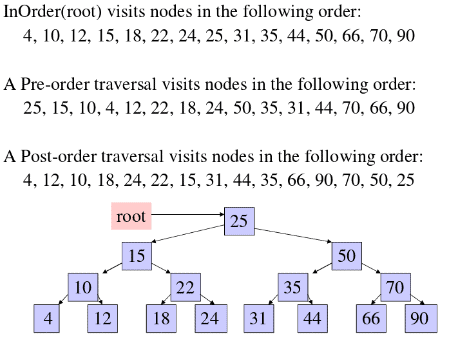Time Complexity: O(n)
Let us see different corner cases.
Complexity function T(n) — for all problem where tree traversal is involved — can be defined as:

T(n) = T(k) + T(n – k – 1) + c

Where k is the number of nodes on one side of root and n-k-1 on the other side.

Let’s do analysis of boundary conditions

Case 1: Skewed tree (One of the subtrees is empty and other subtree is non-empty)

k is 0 in this case.
T(n) = T(0) + T(n-1) + c
T(n) = 2T(0) + T(n-2) + 2c
T(n) = 3T(0) + T(n-3) + 3c
T(n) = 4T(0) + T(n-4) + 4c

…………………………………………
………………………………………….
T(n) = (n-1)T(0) + T(1) + (n-1)c
T(n) = nT(0) + (n)c

Value of T(0) will be some constant say d. (traversing a empty tree will take some constants time)

T(n) = n(c+d)
T(n) = Θ(n) (Theta of n)

Case 2: Both left and right subtrees have equal number of nodes.

T(n) = 2T(|_n/2_|) + c

## BFS vs DFS for Binary TreeWhat are BFS and DFS for Binary Tree?

A Tree is typically traversed in two ways:

• Breadth First Traversal (Or Level Order Traversal)
• Depth First Traversals
• Inorder Traversal (Left-Root-Right)
• Preorder Traversal (Root-Left-Right)
• Postorder Traversal (Left-Right-Root)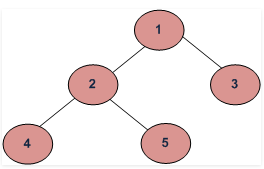BFS and DFSs of above Tree

Breadth First Traversal : 1 2 3 4 5

Depth First Traversals:
Preorder Traversal : 1 2 4 5 3
Inorder Traversal  :  4 2 5 1 3
Postorder Traversal : 4 5 2 3 1

Why do we care?

There are many tree questions that can be solved using any of the above four traversals.

Is there any difference in terms of Time Complexity?

All four traversals require O(n) time as they visit every node exactly once.

Is there any difference in terms of Extra Space?

There is difference in terms of extra space required.

1. Extra Space required for Level Order Traversal is O(w) where w is maximum width of Binary Tree. In level order traversal, queue one by one stores nodes of different level.
2. Extra Space required for Depth First Traversals is O(h) where h is maximum height of Binary Tree. In Depth First Traversals, stack (or function call stack) stores all ancestors of a node.

Maximum Width of a Binary Tree at depth (or height) h can be 2h where h starts from 0. So the maximum number of nodes can be at the last level. And worst case occurs when Binary Tree is a perfect Binary Tree with numbers of nodes like 1, 3, 7, 15, …etc. In worst case, value of 2h is Ceil(n/2).

Height for a Balanced Binary Tree is O(Log n). Worst case occurs for skewed tree and worst case height becomes O(n).

So in worst case extra space required is O(n) for both. But worst cases occur for different types of trees.

It is evident from above points that extra space required for Level order traversal is likely to be more when tree is more balanced and extra space for Depth First Traversal is likely to be more when tree is less balanced.

How to Pick One?

1. Extra Space can be one factor (Explained above)
2. Depth First Traversals are typically recursive and recursive code requires function call overheads.
3. The most important points is, BFS starts visiting nodes from root while DFS starts visiting nodes from leaves. So if our problem is to search something that is more likely to closer to root, we would prefer BFS. And if the target node is close to a leaf, we would prefer DFS.
Offer running on EduRev: Apply code STAYHOME200 to get INR 200 off on our premium plan EduRev Infinity!

## GATE Computer Science Engineering(CSE) 2022 Mock Test Series

131 docs|159 tests

,

,

,

,

,

,

,

,

,

,

,

,

,

,

,

,

,

,

,

,

,

;